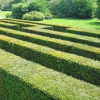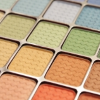#### You may also likeThis comes in two parts, with the first being less fiendish than the second. Itâ€™s great for practising both quadratics and laws of indices, and you can get a lot from making sure that you find all the solutions. For a real challenge (requiring a bit more knowledge), you could consider finding the complex solutions.### Discriminating

You're invited to decide whether statements about the number of solutions of a quadratic equation are always, sometimes or never true.This will encourage you to think about whether all quadratics can be factorised and to develop a better understanding of the effect that changing the coefficients has on the factorised form.

# Two-way Functions

##### Age 16 to 18This resource is from Underground Mathematics.

Each column and row heading in the following table is a property that a function may or may not have.  A function can appear in a cell if it has the properties in the corresponding row and column.

We have omitted some headings, and some entries in cells.  Can you complete the table?

You might find it helpful to draw some sketches.  You could use graph-sketching software such as Desmos to help you, but try to do the sketching by hand first, before reaching for a computer or calculator!

Make sure that you can explain why each function has the desired properties.

$y$-axis is an asymptote     passes through origin
$x = 1$ is a root   $y = \vert x-1\vert$ $y = -3x+3$
has exactly two roots   $y = x(x-2)$
$y = \dfrac{1}{x}$ for $x \neq 0$ $y = \dfrac{1}{(x-1)^2}$ for $x \neq 1$ $y = \dfrac{3}{x+1}$ for $x \neq -1$
$y \to \infty$ as $x \to \infty$     $y = 2+(x-1)^4$

Can you complete the table using a different function in every cell?  By contrast, how few different functions can you use in the table?

This is an Underground Mathematics resource.

Underground Mathematics is hosted by Cambridge Mathematics. The project was originally funded by a grant from the UK Department for Education to provide free web-based resources that support the teaching and learning of post-16 mathematics.

Visit the site at undergroundmathematics.org to find more resources, which also offer suggestions, solutions and teacher notes to help with their use in the classroom.